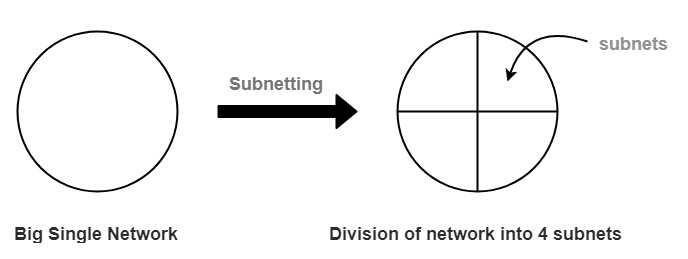# What are subnets and subnet masks in computer networks?

Subnet Mask is used in networking to create multiple sub networks in a network. It divides the IP address into multiple parts which can be assigned to every computer. Subnet Mask is made by setting the network bits to all "1"s and setting host bits to all "0"s. Within a given network, two host addresses are reserved for a special purpose, and cannot be assigned to hosts.

The binary "0" in the subnet mask tell us about the host address. It tells us about the IP of the host which has done subnetting.

## Example

Suppose we have a subnet address as 192.168.1.0. In this, all host addresses are zero. It will be represented in binary as

11000000 10101000 00000001 00000000

In this way, we set the host bits to zero in the Subnet mask.

## Subnet (sub network)

Every website needs a unique IP address, in order to uniquely identify the website, we are dividing the IP network into two or more networks called subnet, which is preferred to control network traffic.

It is a smaller network inside a large network. This technique makes the network routing an efficient one.

## Features

The main features of subnet are as follows −

• Reduce network congestion.
• Control network growth
• Boost network security

Generally, we divide the network into smaller networks or subnets which is called subnetting.

## Use of dividing the network

Let’s take a class A of network. In class A, we have 224hosts. To manage such a large number of hosts is a very difficult job. So, if we divide this large network into the smaller network then maintaining each network would become easy.

Given below is the diagram of subnet −